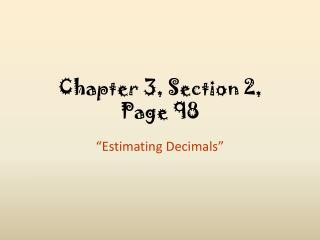DownloadDownload PresentationChapter 3, Section 2, Page 98

# Chapter 3, Section 2, Page 98

Download Presentation## Chapter 3, Section 2, Page 98

- - - - - - - - - - - - - - - - - - - - - - - - - - - E N D - - - - - - - - - - - - - - - - - - - - - - - - - - -
##### Presentation Transcript

1. Chapter 3, Section 2, Page 98 “Estimating Decimals”

2. Warm Up Order the decimals from least to greatest. 1. 18.74, 18.7, 18.47 2. 9.06, 9.66, 9.6, 9.076 Write each in words. 3. 3.072 4. 6.1258 18.47, 18.7, 18.74 9.06, 9.076, 9.6, 9.66 three and seventy-two thousandths six and one thousand two hundred fifty-eight ten-thousandths

3. Sunshine State Standards MA.6.A.5.2 Estimate the results of computations with…decimals…and judge the reasonableness of the results.

4. Vocabulary You Need to Know When numbers are about the same value, you can use clustering to estimate. Clustering means rounding the numbers to the same value. Compatible numbers are close to the numbers that are in the problem and are helpful when you are solving the problem mentally.

5. The addends cluster around 170. To estimate the total number of calories, round each addend to 170. Add. Example 1: Health Application Nancy wants to cycle, ice skate, and water ski for 30 minutes each. About how many calories will she burn in all? (Cycling = 165.5 calories, ice skating = 177.5 calories, and water skiing = 171.5 calories) 165.5 170 177.5 170 171.5 + 170 510 Nancy burns about 510 calories.

6. The addends cluster around 190. To estimate the total number of calories, round each addend to 190. Add. Check It Out: Example 1 Abner wants to run, roller skate, and snow ski for 60 minutes each. About how many calories will he burn in all? (Running = 185.5 calories, roller skating = 189.5 calories, and snow skiing = 191.5 calories) 185.5 190 189.5 190 191.5 + 190 570 Abner burns about 570 calories.

7. Caution! • Look at the digit to the right of the place to which you are rounding. • If it is 5 or greater, round up. • If it is less than 5, round down. 5 or more, raise the score! 4 or less, let it rest!

8. Round to the nearest whole number. The sum is about 12. Round to the tenths. Align. – 0.2683 –0.3 Subtract. Rounding Decimals to Estimate Sums and Differences Estimate by rounding to the indicated place value. A. 7.13 + 4.68; ones B. 9.705 – 0.2683; tenths 7.13 + 4.68 7 + 5 = 12 9.705 9.7 9.4

9. Round to the nearest whole number. The sum is about 10. Round to the tenths. Align. –0.4619 –0.5 Subtract. Example 2 Estimate by rounding to the indicated place value. A. 6.09 + 3.72; ones B. 8.898 – 0.4619; tenths 6.09 + 3.72 6 + 4 = 10 8.898 8.9 8.4

10. 35 and 2 are compatible. 75 and 25 are compatible. Using Compatible Numbers to Estimate Products and Quotients Estimate each product or quotient. A. 33.83  1.98 B. 72.77 ÷ 26.14 352 = 70 So 33.83  1.98 is about 70. 75 ÷ 25 = 3 So, 72.77 ÷ 26.14 is about 3.

11. 20 and 5 are compatible. 60 and 20 are compatible. Example 3 Estimate each product or quotient. A. 22.12  4.98 B. 62.31 ÷ 18.52 205 = 100 So 22.12  4.98 is about 100. 60 ÷ 20 = 3 So, 62.31 ÷ 18.52 is about 3.

12. You can also use front-end estimationto estimate with decimals. Front-end estimation means to use only the whole-number part of the decimal. No Rounding Here

13. Add the whole numbers only. The whole-number values of the decimals are less than the actual numbers, so the answer is an underestimate. Using Front-End Estimation Estimate a range for the sum. 7.86 + 36.97 + 5.40 Use front-end estimation. 7.86 7 36.97 36 5.40 + 5 at least 48 The exact answer of 7.86 + 36.97 + 5.40 is greater than 48.

14. Add the adjusted decimal part of the numbers. Add the whole-number estimate and this sum. The adjusted decimals are greater than the actual decimal, so 50.50 is an overestimate. Example 4 Continued You can estimate a range for the sum by adjusting the decimal part of the numbers. Round the decimals up to 0.5 or 1. 0.86 1.00 0.97 1.00 + 0.50 0.40 2.50 48.00 + 2.50 = 50.50 The estimated range for the sum is from 48.00 to 50.50.

15. Add the whole numbers only. The whole-number values of the decimals are less than the actual numbers, so the answer is an underestimate. Example Estimate a range for the sum. 8.92 + 47.88 + 3.41 Use front-end estimation. 8.92 8 47.88 47 3.41 + 3 at least 58 The exact answer of 8.92 + 47.88 + 3.41 is greater than 58.

16. Lesson Quiz Estimate by rounding to the indicated place value. 1. 3.07442 + 1.352; tenths 2. 7.305 – 4.12689; nearest whole number 4.5 3 Estimate each product or quotient. 14 3. 6.75  1.82 3 4. 10.5 ÷ 3.42 5. The snowfall in December, January, and February was 18.26 cm, 29.36 cm, and 32.87 cm, respectively. About how many total centimeters of snow fell during the three months? 80 cm

17. HOMEWORK Class Periods 1, 4 & 7 Page 100, #12 – 24 EVEN PROBLEMS ONLY (12, 14, 16, 18, 20, 22, 24) Class Periods 2, 3 & 6 (Advanced) Page 100, #12 – 24 EVEN PROBLEMS ONLY (12, 14, 16, 18, 20, 22, 24) AND #27 & 28